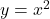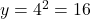## Select the correct answer. x y 2.5 6.25 9.4 88.36 15.6 243.63 19.5 380.25 25.8 665.64 The table lists the values for two parameters, x and y

Question

Select the correct answer. x y 2.5 6.25 9.4 88.36 15.6 243.63 19.5 380.25 25.8 665.64 The table lists the values for two parameters, x and y, of an experiment. What is the approximate value of y for x = 4? A. 11 B. 16 C. 24 D. 43

in progress 0
5 months 2021-08-09T14:28:03+00:00 2 Answers 26 views 0

Explanation:

The given table:

x y

2.5 6.25

9.4 88.36

15.6 243.63

19.5 380.25

25.8 665.64

Here, we observed that value of y is the square value of x.

i.e.[For example 2.5²=6.25, 9.4²=88.36, 15.6²=243.63]

Put x=4, we getHence, the approximate value of y for x = 4 is 16.

So, the correct option is B. 16 .